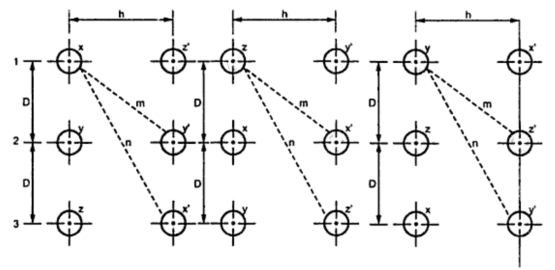### Inductance of Three Phase Double Circuit with Unsymmetrical Spacing but Transposed

Consider three phase double circuit having conductors which are Unsymmetrically spaced. The line is transposed so that each conductor occupies the position of other conductor after certain interval length or distance of transmission line.
The transposition cycle is as shown in the Fig.1.Fig. 1

Flux linkage of conductor x in position 1
Similarly,
As       Ix + Iy + Iz = 0          ...   I = - (Iy + Iz )
Inductance of each phase = 0.5 Inductance per conductor